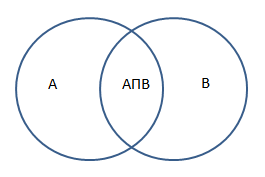### Intersection of Set(A Intersect B) or A∩B Calculation

Set is the relation of some given data or say set contains the elements of similar type or category. A set is defined as the collection of elements, such as numbers or other objects, which are arranged in a group. The set with any numbers can be denoted in the symbol braces { }. The intersection of the sets A and set B is represented by A ∩ B and it is pronounced as A intersection B.for example: The two sets of events A={1, 2, 3,4}
and B={3,4, 6, 7, 8}
the intersection of the sets we get A ∩ B = {3, 4}
 Enter the values in the set1(seperated by comma) Enter the values in the set2(seperated by comma) Intersection of sets